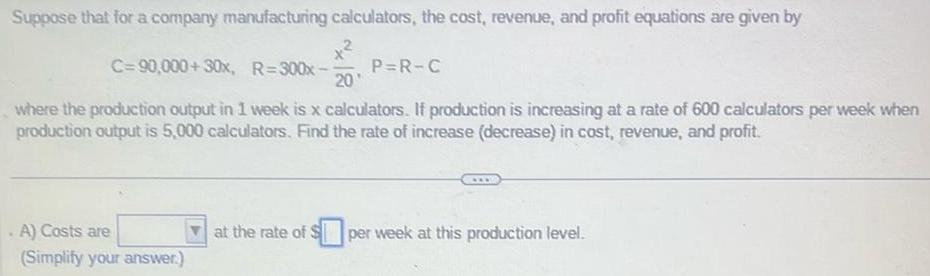Question:

# Suppose that for a company manufacturing calculators the

Last updated: 11/18/2023Suppose that for a company manufacturing calculators the cost revenue and profit equations are given by C 90 000 30x R 300x 20 where the production output in 1 week is x calculators If production is increasing at a rate of 600 calculators per week when production output is 5 000 calculators Find the rate of increase decrease in cost revenue and profit A Costs are Simplify your answer P R C at the rate of S per week at this production level